# Data Wrangling in Python

## Data Wrangling in Python

Data Wrangling is the process of gathering, collecting, and transforming Raw data into another format for better understanding, decision-making, accessing, and analysis in less time. Data Wrangling is also known as Data Munging.## Importance Of Data Wrangling

Data Wrangling is a very important step. The below example will explain its importance as :

Books selling Website want to show top-selling books of different domains, according to user preference. For example, a new user search for motivational books, then they want to show those motivational books which sell the most or having a high rating, etc.

But on their website, there are plenty of raw data from different users. Here the concept of Data Munging or Data Wrangling is used. As we know Data is not Wrangled by System. This process is done by Data Scientists. So, the data Scientist will wrangle data in such a way that they will sort that motivational books that are sold more or have high ratings or user buy this book with these package of Books, etc. On the basis of that, the new user will make choice. This will explain the importance of Data wrangling.

## Data Wrangling in Python

Data Wrangling is a crucial topic for Data Science and Data Analysis. Pandas Framework of Python is used for Data Wrangling. Pandas is an open-source library specifically developed for Data Analysis and Data Science. The process like data sorting or filtration, Data grouping, etc.

Data wrangling in python deals with the below functionalities:

1. Data exploration: In this process, the data is studied, analyzed and understood by visualizing representations of data.
2. Dealing with missing values: Most of the datasets having a vast amount of data contain missing values of NaN, they are needed to be taken care of by replacing them with mean, mode, the most frequent value of the column or simply by dropping the row having a NaN value.
3. Reshaping data: In this process, data is manipulated according to the requirements, where new data can be added or pre-existing data can be modified.
4. Filtering data: Some times datasets are comprised of unwanted rows or columns which are required to be removed or filtered
5. Other: After dealing with the raw dataset with the above functionalities we get an efficient dataset as per our requirements and then it can be used for a required purpose like data analyzing, machine learning, data visualization, model training etc.

#### Below is an example which implements the above functionalities on a raw dataset:

• Data exploration, here we assign the data, and then we visualize the data in a tabular format.
 `# Import pandas package` `import` `pandas as pd` `# Assign data` `data ``=` `{``'Name'``: [``'Jai'``, ``'Princi'``, ``'Gaurav'``,` `                 ``'Anuj'``, ``'Ravi'``, ``'Natasha'``, ``'Riya'``],` `        ``'Age'``: [``17``, ``17``, ``18``, ``17``, ``18``, ``17``, ``17``],` `        ``'Gender'``: [``'M'``, ``'F'``, ``'M'``, ``'M'``, ``'M'``, ``'F'``, ``'F'``],` `        ``'Marks'``: [``90``, ``76``, ``'NaN'``, ``74``, ``65``, ``'NaN'``, ``71``]}` `# Convert into DataFrame` `df ``=` `pd.DataFrame(data)` `# Display data` `df`

Output: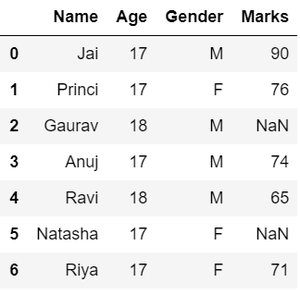• Dealing with missing values, as we can see from the previous output, there are NaN values present in the MARKS column which are going to be taken care of by replacing them with the column mean.
 `# Compute average` `c ``=` `avg ``=` `0` `for` `ele ``in` `df[``'Marks'``]:` `    ``if` `str``(ele).isnumeric():` `        ``c ``+``=` `1` `        ``avg ``+``=` `ele` `avg ``/``=` `c` `# Replace missing values` `df ``=` `df.replace(to_replace``=``"NaN"``,` `                ``value``=``avg)` `# Display data` `df`

Output: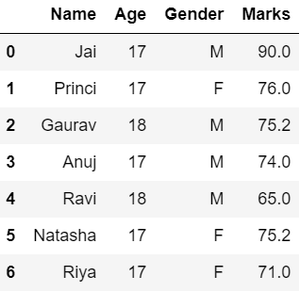• Reshaping data, in the GENDER column, we can reshape the data by categorizing them into different numbers.
 `# Categorize gender` `df[``'Gender'``] ``=` `df[``'Gender'``].``map``({``'M'``: ``0``,` `                                 ``'F'``: ``1``, }).astype(``float``)` `# Display data` `df`

Output: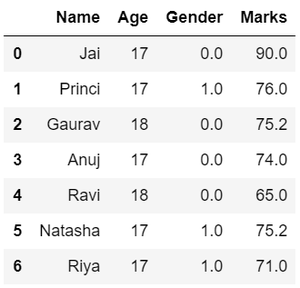• Filtering data, suppose there is a requirement for the details regarding name, gender, marks of the top-scoring students. Here we need to remove some unwanted data.
 `# Filter top scoring students` `df ``=` `df[df[``'Marks'``] >``=` `75``]` `# Remove age row` `df ``=` `df.drop([``'Age'``], axis``=``1``)` `# Display data` `df`

Output: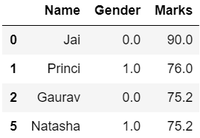Hence, we have finally obtained an efficient dataset which can be further used for various purposes.

Now that we know the basics of data wrangling. Below we will discuss various operations using which we can perform data wrangling:

## Wrangling Data Using Merge Operation

Merge operation is used to merge raw data and into the desired format.

Syntax:

`pd.merge( data_frame1,data_frame2, on="field ")`

Here the field is the name of the column which is similar on both data-frame.

For example: Suppose that a Teacher has two types of Data, first type of Data consist of Details of Students and Second type of Data Consist of Pending Fees Status which is taken from Account Office. So The Teacher will use merge operation here in order to merge the data and provide it meaning. So that teacher will analyze it easily and it also reduces time and effort of Teacher from Manual Merging.

FIRST TYPE OF DATA:

 `# import module` `import` `pandas as pd   ` `# creating DataFrame for Student Details` `details ``=` `pd.DataFrame({` `    ``'ID'``: [``101``, ``102``, ``103``, ``104``, ``105``, ``106``,` `           ``107``, ``108``, ``109``, ``110``],` `    ``'NAME'``: [``'Jagroop'``, ``'Praveen'``, ``'Harjot'``,` `             ``'Pooja'``, ``'Rahul'``, ``'Nikita'``,` `             ``'Saurabh'``, ``'Ayush'``, ``'Dolly'``, ``"Mohit"``],` `    ``'BRANCH'``: [``'CSE'``, ``'CSE'``, ``'CSE'``, ``'CSE'``, ``'CSE'``,` `               ``'CSE'``, ``'CSE'``, ``'CSE'``, ``'CSE'``, ``'CSE'``]})` `# printing details` `print``(details)`

Output: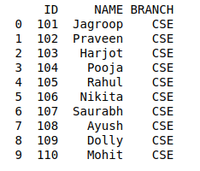SECOND TYPE OF DATA

 `# Import module` `import` `pandas as pd` `# Creating Dataframe for Fees_Status` `fees_status ``=` `pd.DataFrame(` `    ``{``'ID'``: [``101``, ``102``, ``103``, ``104``, ``105``,` `            ``106``, ``107``, ``108``, ``109``, ``110``],` `     ``'PENDING'``: [``'5000'``, ``'250'``, ``'NIL'``,` `                 ``'9000'``, ``'15000'``, ``'NIL'``,` `                 ``'4500'``, ``'1800'``, ``'250'``, ``'NIL'``]})` `# Printing fees_status` `print``(fees_status)`

Output: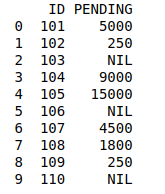WRANGLING DATA USING MERGE OPERATION:

 `# Import module` `import` `pandas as pd` `# Creating Dataframe` `details ``=` `pd.DataFrame({` `    ``'ID'``: [``101``, ``102``, ``103``, ``104``, ``105``,` `           ``106``, ``107``, ``108``, ``109``, ``110``],` `    ``'NAME'``: [``'Jagroop'``, ``'Praveen'``, ``'Harjot'``,` `             ``'Pooja'``, ``'Rahul'``, ``'Nikita'``,` `             ``'Saurabh'``, ``'Ayush'``, ``'Dolly'``, ``"Mohit"``],` `    ``'BRANCH'``: [``'CSE'``, ``'CSE'``, ``'CSE'``, ``'CSE'``, ``'CSE'``,` `               ``'CSE'``, ``'CSE'``, ``'CSE'``, ``'CSE'``, ``'CSE'``]})` `# Creating Dataframe` `fees_status ``=` `pd.DataFrame(` `    ``{``'ID'``: [``101``, ``102``, ``103``, ``104``, ``105``,` `            ``106``, ``107``, ``108``, ``109``, ``110``],` `     ``'PENDING'``: [``'5000'``, ``'250'``, ``'NIL'``,` `                 ``'9000'``, ``'15000'``, ``'NIL'``,` `                 ``'4500'``, ``'1800'``, ``'250'``, ``'NIL'``]})` `# Merging Dataframe` `print``(pd.merge(details, fees_status, on``=``'ID'``))`

Output: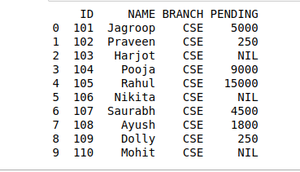## Wrangling Data using Grouping Method

The grouping method in Data analysis is used to provide results in terms of various groups taken out from Large Data. This method of pandas is used to group the outset of data from the large data set.

Example: There is a Car Selling company and this company have different Brands of various Car Manufacturing Company like Maruti, Toyota, Mahindra, Ford, etc. and have data where different cars are sold in different years. So the Company wants to wrangle only that data where cars are sold during the year 2010. For this problem, we use another Wrangling technique that is groupby() method.

CARS SELLING DATA:

 `# Import module` `import` `pandas as pd` `# Creating Data` `car_selling_data ``=` `{``'Brand'``: [``'Maruti'``, ``'Maruti'``, ``'Maruti'``,` `                              ``'Maruti'``, ``'Hyundai'``, ``'Hyundai'``,` `                              ``'Toyota'``, ``'Mahindra'``, ``'Mahindra'``,` `                              ``'Ford'``, ``'Toyota'``, ``'Ford'``],` `                    ``'Year'``:  [``2010``, ``2011``, ``2009``, ``2013``,` `                              ``2010``, ``2011``, ``2011``, ``2010``,` `                              ``2013``, ``2010``, ``2010``, ``2011``],` `                    ``'Sold'``: [``6``, ``7``, ``9``, ``8``, ``3``, ``5``,` `                             ``2``, ``8``, ``7``, ``2``, ``4``, ``2``]}` `# Creating Dataframe of car_selling_data` `df ``=` `pd.DataFrame(car_selling_data)` `# printing Dataframe` `print``(df)`

Output: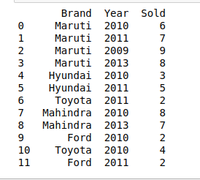DATA OF THE YEAR 2010:

 `# Import module` `import` `pandas as pd` `# Creating Data` `car_selling_data ``=` `{``'Brand'``: [``'Maruti'``, ``'Maruti'``, ``'Maruti'``,` `                              ``'Maruti'``, ``'Hyundai'``, ``'Hyundai'``,` `                              ``'Toyota'``, ``'Mahindra'``, ``'Mahindra'``,` `                              ``'Ford'``, ``'Toyota'``, ``'Ford'``],` `                    ``'Year'``:  [``2010``, ``2011``, ``2009``, ``2013``,` `                              ``2010``, ``2011``, ``2011``, ``2010``,` `                              ``2013``, ``2010``, ``2010``, ``2011``],` `                    ``'Sold'``: [``6``, ``7``, ``9``, ``8``, ``3``, ``5``,` `                             ``2``, ``8``, ``7``, ``2``, ``4``, ``2``]}` `# Creating Dataframe for Provided Data` `df ``=` `pd.DataFrame(car_selling_data)` `# Group the data when year = 2010` `grouped ``=` `df.groupby(``'Year'``)` `print``(grouped.get_group(``2010``))`

Output: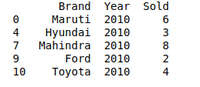## Wrangling data by removing Duplication

Pandas duplicates() method helps us to remove duplicate values from Large Data. An important part of Data Wrangling is removing Duplicate values from the large data set.

Syntax:

`DataFrame.duplicated(subset=None, keep='first')`

Here subset is the column value where we want to remove Duplicate value.

In keep, we have 3 options :

• if  keep =’first’ then the first value is marked as original rest all values if occur will be removed as it is considered as duplicate.
• if keep=’last’ then the last value is marked as original rest all above same values will be removed as it is considered as duplicate values.
• if keep =’false’ the all the values which occur more than once will be removed as all considered as a duplicate value.

For example, A University will organize the event. In order to participate Students have to fill their details in the online form so that they will contact them. It may be possible that a student will fill the form multiple time. It may cause difficulty for the event organizer if a single student will fill multiple entries. The Data that the organizers will get can be Easily Wrangles by removing duplicate values.

DETAILS STUDENTS DATA WHO WANT TO PARTICIPATE IN THE EVENT:

 `# Import module` `import` `pandas as pd` `# Initializing Data` `student_data ``=` `{``'Name'``: [``'Amit'``, ``'Praveen'``, ``'Jagroop'``,` `                         ``'Rahul'``, ``'Vishal'``, ``'Suraj'``,` `                         ``'Rishab'``, ``'Satyapal'``, ``'Amit'``,` `                         ``'Rahul'``, ``'Praveen'``, ``'Amit'``],` `                ``'Roll_no'``: [``23``, ``54``, ``29``, ``36``, ``59``, ``38``,` `                            ``12``, ``45``, ``34``, ``36``, ``54``, ``23``],` `                ``'Email'``: [``'xxxx@gmail.com'``, ``'xxxxxx@gmail.com'``,` `                          ``'xxxxxx@gmail.com'``, ``'xx@gmail.com'``,` `                          ``'xxxx@gmail.com'``, ``'xxxxx@gmail.com'``,` `                          ``'xxxxx@gmail.com'``, ``'xxxxx@gmail.com'``,` `                          ``'xxxxx@gmail.com'``, ``'xxxxxx@gmail.com'``,` `                          ``'xxxxxxxxxx@gmail.com'``, ``'xxxxxxxxxx@gmail.com'``]}` `# Creating Dataframe of Data` `df ``=` `pd.DataFrame(student_data)` `# Printing Dataframe` `print``(df)`

Output: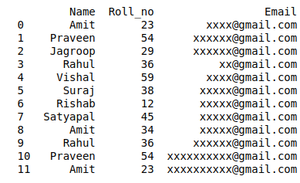DATA WRANGLED BY REMOVING DUPLICATE ENTRIES:

 `# import module` `import` `pandas as pd` `# initializing Data` `student_data ``=` `{``'Name'``: [``'Amit'``, ``'Praveen'``, ``'Jagroop'``,` `                         ``'Rahul'``, ``'Vishal'``, ``'Suraj'``,` `                         ``'Rishab'``, ``'Satyapal'``, ``'Amit'``,` `                         ``'Rahul'``, ``'Praveen'``, ``'Amit'``],` `                ``'Roll_no'``: [``23``, ``54``, ``29``, ``36``, ``59``, ``38``,` `                            ``12``, ``45``, ``34``, ``36``, ``54``, ``23``],` `                ``'Email'``: [``'xxxx@gmail.com'``, ``'xxxxxx@gmail.com'``,` `                          ``'xxxxxx@gmail.com'``, ``'xx@gmail.com'``,` `                          ``'xxxx@gmail.com'``, ``'xxxxx@gmail.com'``,` `                          ``'xxxxx@gmail.com'``, ``'xxxxx@gmail.com'``,` `                          ``'xxxxx@gmail.com'``, ``'xxxxxx@gmail.com'``,` `                          ``'xxxxxxxxxx@gmail.com'``, ``'xxxxxxxxxx@gmail.com'``]}` `# creating dataframe` `df ``=` `pd.DataFrame(student_data)` `# Here df.duplicated() list duplicate  Entries in ROllno.` `# So that ~(NOT) is placed in order to get non duplicate values.` `non_duplicate ``=` `df[~df.duplicated(``'Roll_no'``)]` `# printing non-duplicate values` `print``(non_duplicate)`

Output: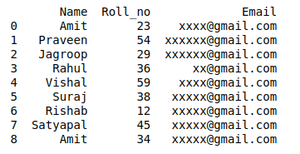Last Updated on October 24, 2021 by admin

## Normalize A Column In PandasNormalize A Column In Pandas

Normalize A Column In Pandas In this article, we will learn how to normalize a

## Python – Extract words from given stringPython – Extract words from given string

Python | Extract words from given string We sometimes come through situations where we require

## pandas.merge_asof() function in Pythonpandas.merge_asof() function in Python

pandas.merge_asof() function in Python This method is used to perform an asof merge. This is

## Apply function to every row in a Pandas DataFrameApply function to every row in a Pandas DataFrame

Apply function to every row in a Pandas DataFrame   Python is a great language

## How to extract date from Excel file using Pandas?How to extract date from Excel file using Pandas?

How to extract date from Excel file using Pandas? In this article, Let’s see how

## How to extract the value names and counts from value_counts() in Pandas ?How to extract the value names and counts from value_counts() in Pandas ?

How to extract the value names and counts from value_counts() in Pandas ? In this

## Python Program for Sum the digits of a given numberPython Program for Sum the digits of a given number

Python Program for Sum the digits of a given number Given a number and the

## Python – Pandas.pivot()Python – Pandas.pivot()

Python | Pandas.pivot() pandas.pivot(index, columns, values) function produces pivot table based on 3 columns of